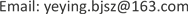1北京市市政工程研究院，北京

2地下工程建设预报预警北京市重点实验室，北京

3北京交通大学城市地下工程教育部重点实验室，北京1. 引言

2. 瞬变电磁法探测混凝土结构可行性

2.1. 探测混凝土结构的原理

Physical parameters related to the concrete structur

800.50.0330.1

2.2. 浅层瞬变电磁“感应–衰减”过程

2.3. 影响浅层瞬变电磁探测的因素

1) 仪器发射机关断时间不够小，采样率不够高；

2) 线圈的暂态过程(过渡过程)：早期一次场与二次场的叠加；

3) 仪器的抗干扰能力和环境噪声的矛盾；

4) 地质体的电性、埋深、几何参数与地电断面的性质。

3. 瞬变电磁结构雷达研制

3.1. 瞬变电磁雷达系统

TER瞬变电磁雷达  由北京市市政工程研究院研制，TER系统由电磁发射机、电磁接收机、天线及处理软件组成。具体指标参见表2。

Transient electromagnetic structure radar technical inde

3.2. “欠阻尼”中心回线装置

d 2 V ( t ) d t 2 + 2 δ d V ( t ) d t + ω 2 V ( t ) = 1 L C ε ( t ) (1)

g ( s ) = 1 L C ( s 2 + 2 K ω s + ω 2 ) (2)

g ( t ) = L − 1 [ g ( s ) ] = { 1 L C α e − ξ s h ( α t ) , ( ξ ≥ 1 ) 1 L C α e − ξ s h ( α t ) , ( ξ < 1 ) (3)

V ( t ) = g ( t ) × ε ( t ) (4)

δ = ( r C + L / R ) / 2 L C

r为瞬变电磁电路系统中接收线圈Rx内阻；

R为外接电阻即配置电阻；L为接收线圈电感；

C为系统中接收线圈的分布电容；

ω = ( r / R + 1 ) / L C 为接收线圈的谐振频率；

α = ω | K 2 − 1 | ，当K < 1、K = 1、K > 1，接收系统分别处于欠阻尼、临界阻尼和过阻尼状态，不同

3.3. 全期ρ<sub>i</sub>视电阻率计算

U i ( t ) = I ρ a 3 [ 3 e r f ( u ) − 2 π u ( 3 + 2 u 2 ) e − u 2 ] (5)

B z i = I μ 0 2 a [ 3 π u e − u 2 + ( 1 − 3 2 u 2 ) e r f ( u ) ] (6)

ρ i ( t ) = μ 0 a 2 4 t u 2 (9)

3.4. 拟地震波谱显示

TER瞬变电磁雷达软件设计了常见地震波各种波形图，分别有波形曲线见图11、波型变密度见图12、等值线见图13、变密度加等值线见图14。图形设计主要便于同相轴追踪、结构异常比较与辨识  。

4. 混凝土结构探测试验

4.1. 楼板测试

4.2. 隧道衬砌试验

5. 结论